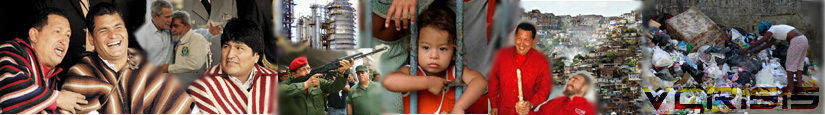# home | Archive | analysis | videos | data | weblognews in other languages: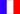fr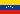esp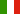ita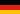de## Benford's Law proves fraud in Venezuela's referendum

### By Miguel Octavio

06.10.04 - I have talked about Benfords law and its prediction as well as quoted results in previous posts, but today I finally received the green light to talk about the details of the work by Pericchi and Torres which you can find in detail here.

Recall that Benfords law or Necomb-Benfords Law (NB) applies to the distribution of first and second digits in a table of numbers. That is, if you take a sample of numbers from many natural populations, the first or second digit are usually not evenly distributed, but follow the following equations for the frequency of their occurrence:

Prob(1st digit = d) = log10(1 + d-1); d = 1, . . . , 9

For the first digit, and:

Prob(2nd. digit=d) = Sum (k=1 to 9)( log10(1 + (10k + d)-1)); d = 0, 1, . . . , 9

For the second digit.

What Pericchi and Torres have done is to check for the NB behavior for the first and second digit in the results of the August 15th. recall vote for both automated and manual votes. They concentrate their analysis on the distribution of second digits because it is not affected by limited ranges of numbers. For example, if one studies the first digit and no voting machine had more than 600 Si or No votes, there will be fewer first digits from 7 to 9, since the only contributing ones would be those from 70 to 99.

The first figure below shows the comparison for both the manual (Top) and automated (Bottom) results for the SI vote for the second digit of all voting notebooks in the recall vote.Figure 1. Manual (Top) and Automated (Bottom) results for the second digit of all the voting results for the total of Si votes in each notebook. The smooth line in both cases is the theoretical value for the NB law and the broken line is the results of analyzing the recall data.

Note that in the case of the Si vote, the data from the recall vote closely follows what is expected from the NB law. In fact, as will be shown below the results are probable.

However, the results are quite different for the No vote as shown below:Figure 2. Manual (Top) and Automated (Bottom) results for the second digit of all the voting results for the total of NO votes in each notebook. The smooth line in both cases is the theoretical value for the NB law and the broken line is the results of analyzing the recall data.

In the case of the NO results while the comparison is quite reasonable for the manual notebooks, the same can not be said for the automated machines where essentially a flat distribution of second digits was obtained, much different than what is expected from BNs law and quite different from Figure 1. for the Si vote .

In fact, one can do exactly the same analysis to the total number of votes per machine SI+No and one finds the following behavior:Figure 3. Manual (Top) and Automated (Bottom) results for the second digit of all the voting results for the total of SI+NO votes in each notebook. The smooth line in both cases is the theoretical value for the NB law and the broken line is the results of analyzing the recall data.

In the case of the total number of votes, once again there are very important discrepancies between the predictions of the BN law and the results.

What Pericchi and Torres did then, was to say that the null hypothesis Ho is that which assumes there was no tampering of the data. They then calculate both the Pvalue and the probability of the occurrences of the data observed assuming no tampering or intervention occurred.

The Pvalue is defined as the probability that a result like the one measured or more extreme is obtained given the null hypothesis, i.e. assuming there was no intervention. Pericchi and Torres then calculate also what is the approximate probability according to the Bayesian Information Criteria (BIC) which takes into account the size of the sample.

The results for all cases are shown in the table below:Table I. Evidence against the null hypothesis Ho. The data follows the Newcomb Benford law, except for the case of the automated No votes. But the manual Si and NO do follow as well as the results of the audit.

What is most remarkable about the quoted results is that the approximate probability that the measured result was obtained for the No vote is 1.34 10-36 ( a one followed by 36 zeroes!). Thus, the probability that the results were not tampered with is simply miniscule or extremely improbable, the NB law is violated and one should think more about how the intervention of the data may have occurred. In my mind this proves fraud, because there is simply no way of explaining these results.

Even more remarkable, which is quoted in the table above, is the fact that similar plots for the audited results on the cold audit performed on Aug. 18th. show that they do follow the BN law:Figure 4. Si (Top) and No (Bottom) results for the second digit of the audited results. The smooth line in both cases is the theoretical value for the NB law and the broken line is the results of analyzing the recall data.

Thus, the audited results for the Si and the No follow the NB law, despite the much smaller sample size in the case of the audit. Thus, once again, the results from the audit and the actual vote are quite different, indicating not only fraud, but that the sample for the audit was carefully picked! I would like the Carter Center, Taylor, Rubin and Weisbrot to explain away this result. I challenge them to do so!